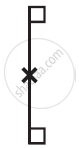# The Following Figure Have Rotational Symmetry of Order More than 1:- 3 - Mathematics

The following figure have rotational symmetry of order more than 1:#### Solution

The given figure has its rotational symmetry as 1

Is there an error in this question or solution?
Chapter 14: Symmetry - Exercise 14.2 [Page 274]

#### APPEARS IN

NCERT Class 7 Maths
Chapter 14 Symmetry
Exercise 14.2 | Q 1.3 | Page 274

Share